# Class 6 Maths NCERT Solutions for Chapter 14 Practical Geometry Ex – 14.5

## Practical Geometry

Question 1.
Draw$\overline { AB }$ of length 7.3 cm and find its axis of symmetry.

Solution:
Steps of Construction:

1. Draw a line segment AB = 7.3 cm.
2. With centre A and radius >$\frac { 1 }{ 2 }$ AB, draw arcs one on each side of AB.
3. With centre B and the same radius as before, draw arcs cutting the previously drawn arcs at C and D respectively.
4. Join CD intersecting AB at M. Then M bisects the line segment AB as shown.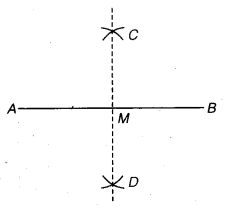The line segment so obtained is the required axis of symmetry.

Question 2.
Draw a line segment of length 9.5 cm and construct its perpendicular bisector.

Solution:
Steps of Construction: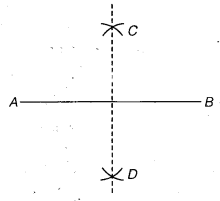1. Draw a line segment AB = 9.5 cm.
2. With centre A and radius >$\frac { 1 }{ 2 }$ AB, draw arcs one on each side of AB. . A
3. With centre B and the same radius as before, draw arcs cutting the previously drawn arcs at C and D respectively.
4. Join CD. Then the line segment CD is the required perpendicular bisector of AB.

Question 3.
Draw the perpendicular bisector of$\overline { XY }$ whose length is 10.3 cm.

(a) Take any point P on the bisector drawn. Examine whether PX = PY.
(b) If M is the mid-point of$\overline { XY }$ , what can you say about the lengths MX and XY?

Solution:
Steps of Construction:

1. Draw a line segment XY =10.3 cm.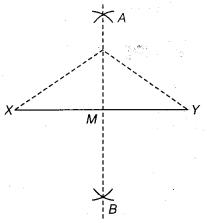2. With centre X and radius >$\frac { 1 }{ 2 }$ XY, draw arcs one on each side of XY.
3. With centre Y and the same radius as before, draw arcs cutting the previously drawn arcs at A and B respectively.
4. oin .AB intersecting XY at M. Then, AB is the perpendicular bisector of XY.
(a) Mark any point P on AB, the perpendicular bisector. On measuring, we find that PX = PY.
(b) Since M is the mid-point of the segment XY. Therefore,
MX =$\frac { 1 }{ 2 }$XY =$\frac { 1 }{ 2 }$ x 10.3 cm
= 5.15 cm

Question 4.
Draw a line segment of length 12.8 cm. Using compasses, divide it into four equal parts. Verify by actual measurement.

Solution:
Steps of Construction: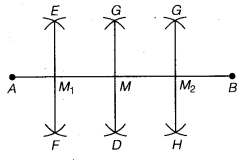1. Draw a line segment AB = 12.8 cm.
2. With centre A and radius >$\frac { 1 }{ 2 }$ AB, draw arcs one on each side of AB.
3. With centre B and the same radius as before, draw arcs cutting the previously drawn arcs at C and D respectively.
4. Join CD intersecting AB at M.
5. Further find the mid-points M1 and M2 of AM and MB respectively proceeding in the same way qs-before.
∴ AM1 = M1M = MM2 = M2B, On measuring, we find that each part = 3.2 cm.

Question 5.
With$\overline { PQ }$ of length 6.1 cm as diameter draw a circle.

Solution:
Steps of Construction: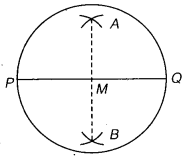1. Draw a line segment PQ = 6.1 cm.
2. Bisect the segment PQ by drawing its perpendicular bisector. Let M be its mid-point.
3. M as centre and radius = MP draw a circle.
The circle so obtained is the required circle.

Question 6.
Draw a circle with centre C and radius 3.4 cm. Draw any chord$\overline { AB }$. Construct the perpendicular bisector of$\overline { AB }$ and examine if it passes through C.

Solution:
Steps of Construction: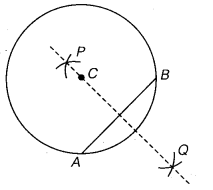1. Mark a point C on the plane of a paper.
2.  With C as centre and radius 3.4 cm, draw a circle.
3. Let AB be any chord to this circle.
4. Draw PQ, the perpendicular bisector of chord AB.
Clearly, this perpendicular bisector passes through C, the centre of the circle.

Question 7.
Repeat Question 6, if AB happens to he a diameter.

Solution:
If AB happens to be a diameter then C will be the mid-point of the diameter AB.

Question 8.
Draw a circle of radius 4 cm. Draw any two of its chords. Construct the perpendicular bisectors of these chords. Where do they meet?

Solution:
Steps of Construction: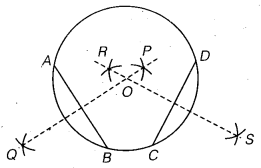1. Mark a point O on the plane of paper.
2. With O as centre, draw a circle of radius 4 cm.
3. Let AB and CD be any two chords of this circle.
4. Draw PQ and RS the perpendicular bisectors of chords AB and CD respectively.
Clearly, these perpendicular bisectors pass through 0, the centre of the circle.

Question 9.
Draw any angle with vertex O. Take a point A on one of its arms and B on another such that OA = OB. Draw the perpendicular bisectors of$\overline { OA }$ and$\overline { OB }$ . Let them meet at P. Is PA = PB?

Solution:
Steps of Construction: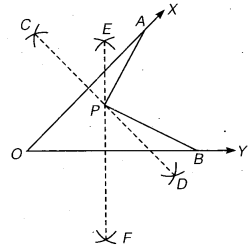1. Draw any angle XOY.
2. Take a point A on OX and a point B on OY such that OA = OB.
3. Draw CD and EF, the perpendicular bisectors of OA and OB respectively. Let them meet at P.
On measuring, we find that PA = PB.